# Alternating current(AC) through pure inductor

## (7) A.C through pure inductor

• Figure below shows the circuit in which voltage source
V=V0sinωt                                  (10)
is applied to pure inductor (zero resistance) coil of inductance L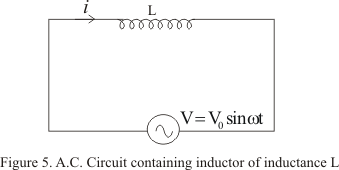• As the current through the inductor varies and opposing induced emf is generated in it and is given by -Ldi/dt
• From Kirchhoff�s loop rule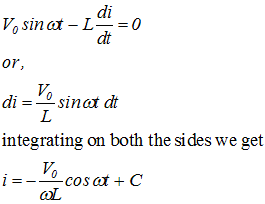Where C is the constant of integration .This integration constant has dimensions of current and is independent of time. Since source has an emf which oscillates symmetrically about zero, the current it sustain also oscillates symmetrically about zero, so there is no time independent component of current that exists. Thus constant C=0
• So we have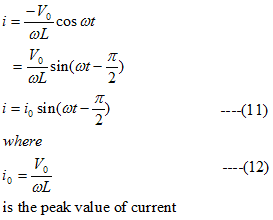• From instantaneous values of current and voltage (equation 11 and 10) we see that in pure inductive circuit the current lags behind emf by a phase angle of π/2
• This phase relationship is graphically shown below in the figure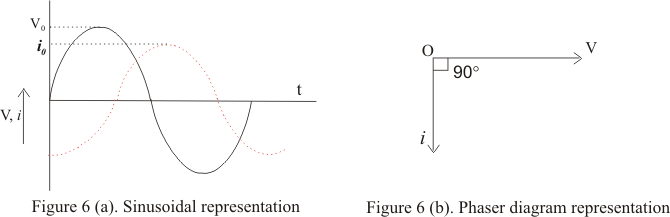• From equation (12) peak value of current in the coil is
i0=V0/ωL
or
V0=(ωL)i0
Comparing it with the ohm's law we find product ωL has dimension of resistance and it can be represented by
XL=ωL
where XL is known as reactance of the coil which represents the effective opposition of the coil to the flow of alternating current
• XL=ωL is zero for DC for which ω=0 and increases as the frequency of current increases

## (8)AC through pure capacitor

• Figure given below shows circuit containing alternating voltage source
V=V0sinωt
connected to a capacitor of capacitance C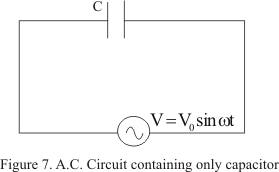• Suppose at any time t,q be the charge on the capacitor and i be the current in the circuit
• Since there is no resistance in the circuit, so the instantaneous potential drop q/C across the capacitor must be equal to applied alternating voltage
so
q/C=V0sinωt
• Since i=dq/dt is the instantaneous current in the circuit so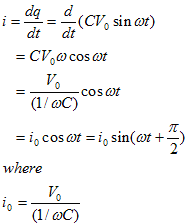is the peak value of current
• Comparing equation (13) with V=V0sinωt ,we see that in a perfect capacitor current leads emf by a phase angle of π/2
• This phase relationship is graphically shown below in the figure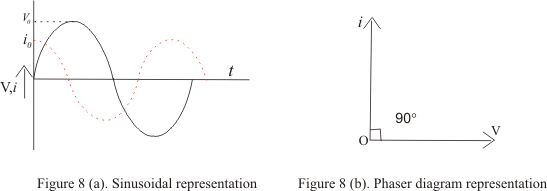• Again comparing peak value of current with ohm's law ,we find that quantity 1/ωC has the dimension of the resistance
• Thus the quantity
XC=1/ωC =1/2πfC ---(14)
is known as capacitive reactance
• From equation (14) we see that capacitive reactance decreases with increasing frequency of current and in infinite for direct current for which frequency f=0

Note to our visitors :-

Thanks for visiting our website.